#sklearn调包侠之线性回归

0
0
01. 云栖社区>
2. 博客>
3. 正文

## sklearn调包侠之线性回归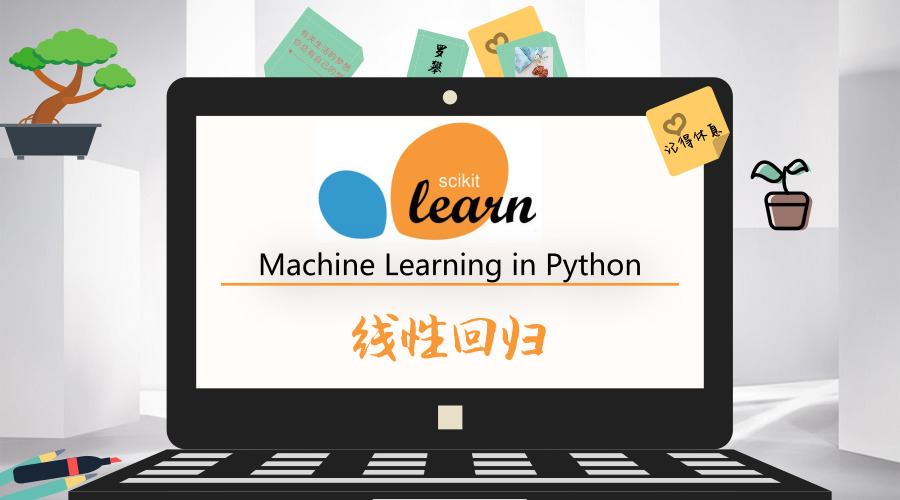### 线性回归原理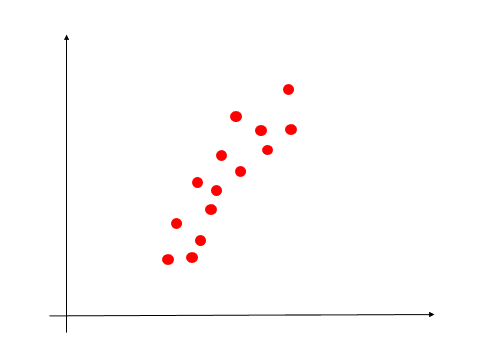##### 目标函数（成本函数）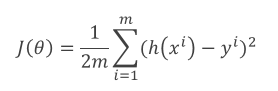##### 梯度下降法

tips：这篇文章讲解了梯度上升法，梯度下降法类似。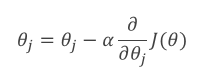### 实战——房价预测

##### 数据导入

``````from sklearn.datasets import load_boston
``````

``````print(boston.DESCR)
``````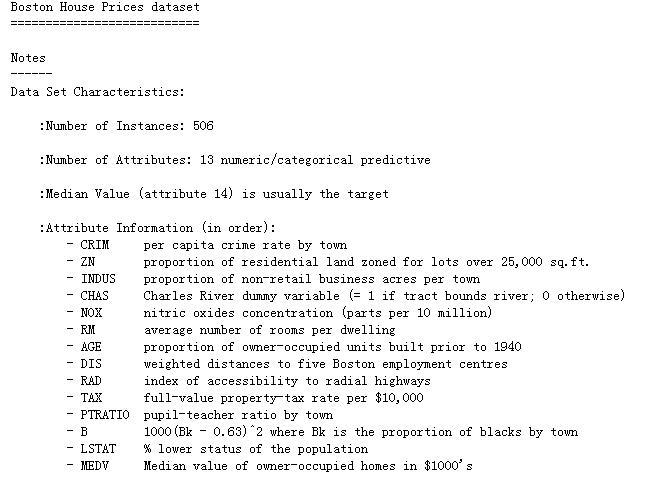boston的data和target分别存储了特征和标签：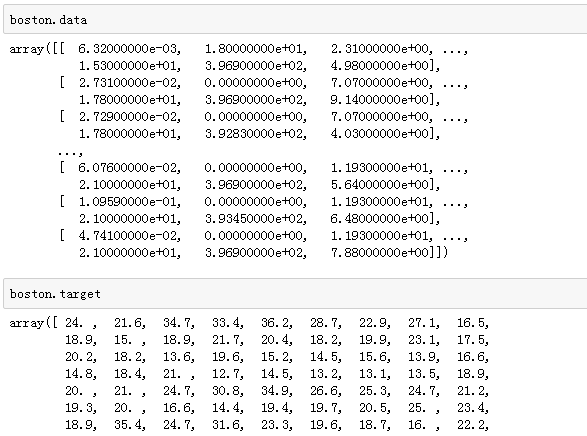##### 切分数据集
``````from sklearn.model_selection import train_test_split
X_train, X_test, y_train, y_test = train_test_split(boston.data, boston.target, test_size = 0.2, random_state=2)
``````
##### 数据预处理

• degree：多项式特征的个数，默认为2
• include_bias：默认为True，包含一个偏置列，也就是 用作线性模型中的截距项，这里选择False，因为在线性回归中，可以设置是否需要截距项。
``````from sklearn.preprocessing import PolynomialFeatures
poly = PolynomialFeatures(degree=2,include_bias=False)
X_train_poly = poly.fit_transform(X_train)
X_test_poly = poly.fit_transform(X_test)
``````
##### 模型训练与评估

• fit_intercept：默认为True，是否计算截距项。
• normalize：默认为False，是否对数据归一化。

``````from sklearn.linear_model import LinearRegression

model2 = LinearRegression(normalize=True)
model2.fit(X_train, y_train)
model2.score(X_test, y_test)

# result
# 0.77872098747725804
``````

``````model3 = LinearRegression(normalize=True)
model3.fit(X_train_poly, y_train)
model3.score(X_test_poly, y_test)

# result
# 0.895848854203947
``````

+ 关注